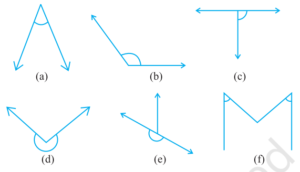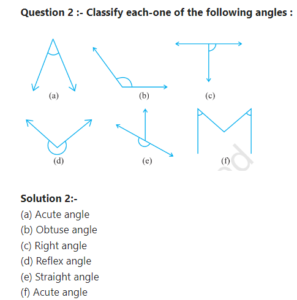# Understanding Elementary Shapes NCERT Solutions for Class 6 Math Exercise 5.3

## Understanding Elementary Shapes Class 6 Math Chapter 5 Ex. 5.3

Understanding Elementary Shapes Ncert Class 6 Math Free Solution.

Exercise 5.3 (Understanding Elementary Shapes )

Question 1 :- Match the following:

(i) Straight angle                      (a) Less than one-fourth of a revolution.
(ii) Right angle                         (b) More than half a revolution.
(iii) Acute angle                       (c) Half of a revolution.
(iv) Obtuse angle                     (d) One-fourth of a revolution.
(v) Reflex angle                        (e) Between 1/4 and 1/2 of a revolution.
(f) One complete revolution.

Solution 1:-
(i) Straight angle          ↔       (c) Half of a revolution.
(ii) Right angle             ↔       (d) One-fourth of a revolution.
(iii) Acute angle            ↔       (a) Less than one-fourth of a revolution.
(iv) Obtuse angle         ↔        (e) Between 1/4 and 1/2 of a revolution.
(v) Reflex angle            ↔        (b) More than half a revolution.

Understanding Elementary Shapes Class 6 Math Solutions Ex.5.3Understanding Elementary Shapes Class 6 Math Solutions Ex.5.3

Question 2 :- Classify each-one of the following angles :Solution 2:-
(a) Acute angle
(b) Obtuse angle
(c) Right angle
(d) Reflex angle
(e) Straight angle
(f) Acute angle

Understanding Elementary Shapes Class 6 Math Solutions Ex.5.3Understanding Elementary Shapes Class 6 Math Solutions Ex.5.3Understanding Elementary Shapes Class 6 Math NCERT Solutions

Understanding Elementary Shapes Class 6 Math Solutions Ex.5.3

Understanding Elementary Shapes Class 6 Math Solutions Ex.5.3

Understanding Elementary Shapes Class 6 Math Solutions Ex.5.3